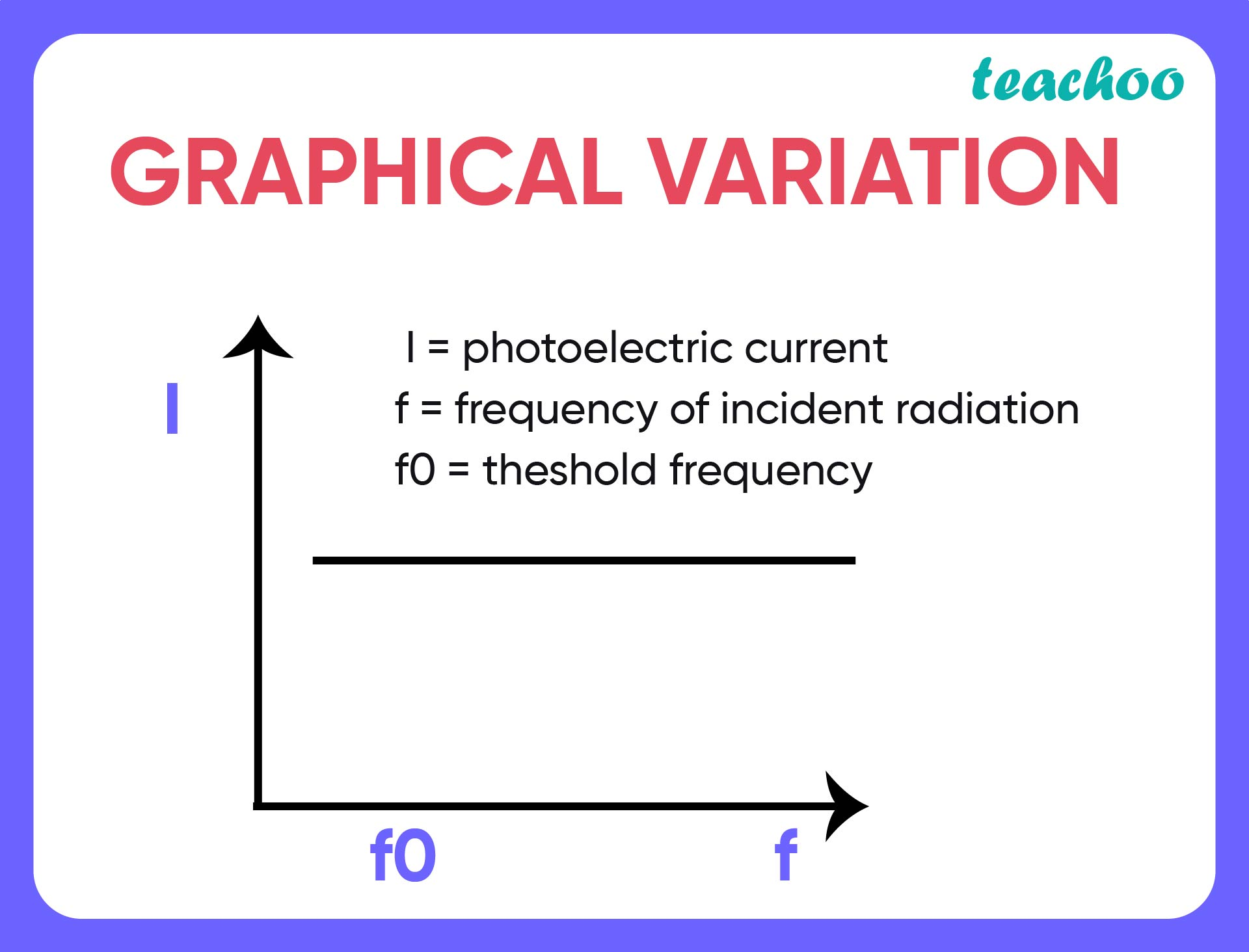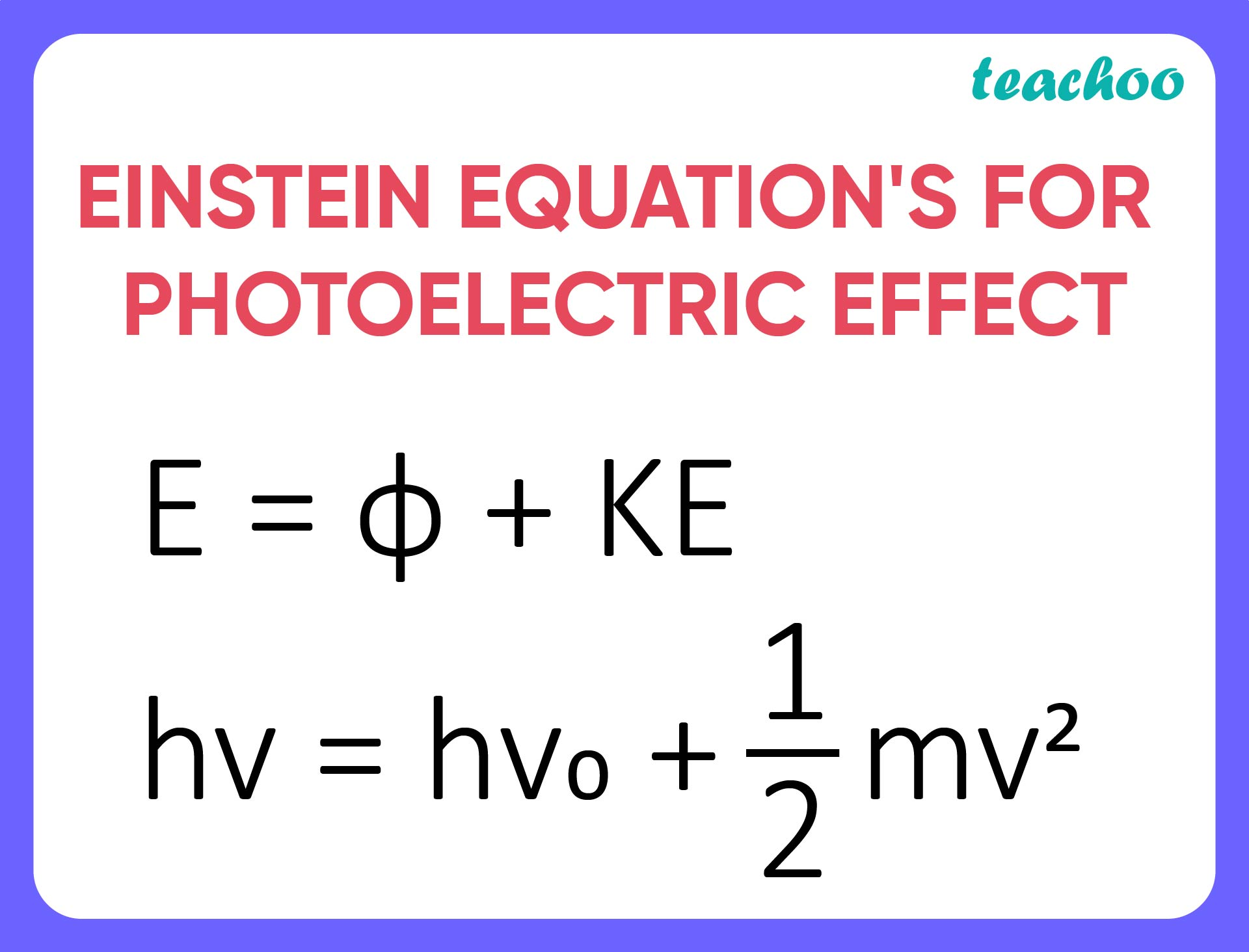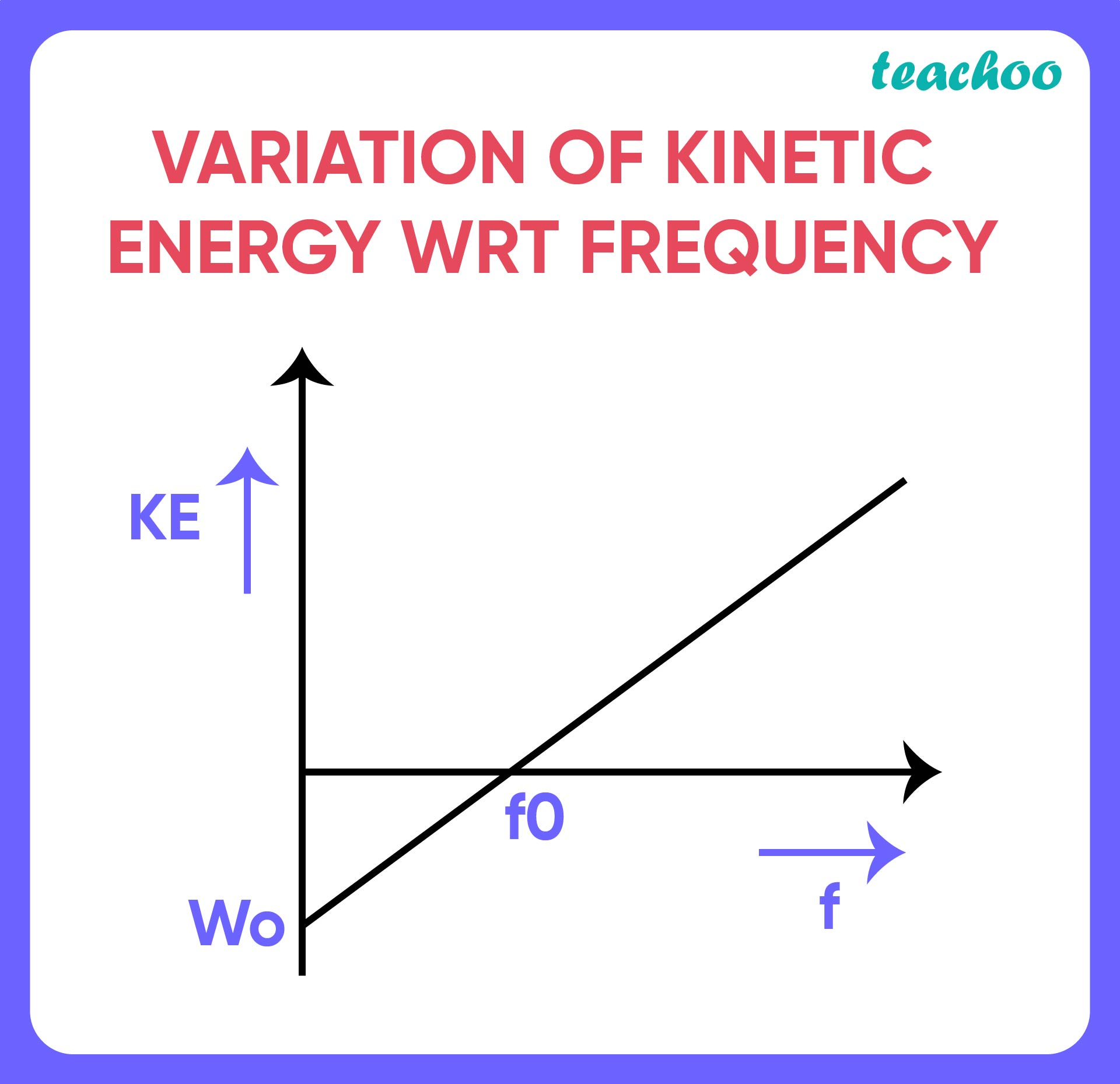CBSE Class 12 Sample Paper for 2022 Boards [Term 2] - Physics

Physics Class 12
Solutions to CBSE Sample Paper - Physics Class 12

## Explain how does (i) photoelectric current and (ii) kinetic energy of the photoelectrons emitted in a photocell vary if the frequency of incident radiation is doubled, but keeping the intensity same? Show the graphical variation in the above two cases.

(i)  Photoelectric current is measured by the number of electrons emitted per second by the metal surface .

• This is entirely dependant on the number of photons that strike the metal surface with frequency above or equal to the threshold frequency.
• It is independent of frequency of the incident photons.(ii)  The variation of kinetic energy with frequency is governed by the Einstein’s Equation for photoelectric effect .Here, KE=hv-hv o

Clearly, Kinetic Energy is linearly dependent on frequency .Hence, the Kinetic energy of photoelectrons emitted would increase with increase in frequency .

Learn in your speed, with individual attention - Teachoo Maths 1-on-1 Class